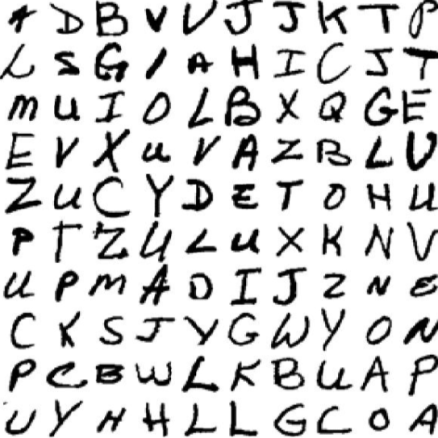CMU

## Neural networkThis is an assignment from CMU 16720-A. In this assignment, I’m going to build a fully connected feed-forward neural network from scratch and then use it to do the handwritten characters classification. In the first part, I will first implement a neural network. In the second part, I will implement a system to locate characters in an image. Then, I will combine them together.

## Implement a fully connected network

Before implementation, I need to answer some theory questions first.

1. Why the ReLU activation function is generally preferred to sigmoid in a deep network?

In a deep network, if we use sigmoid as the activation function, there will be a problem called “vanishing gradient.” This is because the derivative of sigmoid function is , and because is always in the range from 0 to 1, the maximum value of this derivative will be 0.25. When we do the back propagation, the maximum derivative becomes smaller and smaller (0.250.25…), which means the first layer will be very hard to be trained by the output layer. Unlike the sigmoid function, the derivate of ReLU is linear if the input is greater than 0. This is why ReLU is generally preferred.

1. If we have a linear activation function, what would happen?

The network would not be able to express non-linear functions, which means that this network has less expressing power.

1. Why do we use cross-entropy as our loss function?

We use cross-entropy because it’s error surface is steeper compared to square error. For example, if our output is (0.1,0,0.9,0,0) and the ground truth is (1,0,0,0,0), then the error is 0.9^2+0.1^2=0.82. If our output is (0.9,0,0.1,0,0) and ground truth is (1,0,0,0,0), the error is 0.1^2+0.1^2=0.02. Now, if we use cross-entropy, the error will be 3.32 and 0.15 respectively, which means that we can see more difference by using cross-entropy to measure error, and thus we can converge more quickly by using backpropagation.

Now, according to our instruction, we use one hidden layer and one output layer. The activation function for our hidden layer is sigmoid function. We don’t need our activation function in the output layer. Instead, we use a softmax to generate the probability for each category.

For the forward propagation, we just need to multiply the weights and inputs, and then add bias, then sigmoid. In output layer, we just go through softmax, then it’s done. For the backward propagation, we need to find out the partial derivative equation for softmax and sigmoid first, then it’s very easy to implement. Some of the codes are like below.

Then, we can start training our model. Here, I used 30 epochs, and the learning rate was 0.01. After training, the accuracy on test set was 0.8576. There is one thing interesting. I implement the gradient checker. Basically, we randomly select a w in our weights. Then, we add a tiny delta to that weight, and then we do the forward propagation and compute the loss. Then, we reverse w to its original value and then subtract w by delta and do the forward propagation and compute the loss again. Now, we have two loss. We divide the difference of these two losses by 2*delta. This will be our partial derivative with respect to w. Now, reset the w and do a normal forward propagation and backward propagation, we can also get an update value which is based on the partial derivative as well. We can compare these two partial derivatives. If the sign is different, then we might do something wrong. Below is the visualization of weights after we train. Looks like there are some kinds of patterns.

## Extract text from images

I first use the Otsu’s method (graythresh) to do the adaptive thresholding, and then binarize the image. Then, use imcomplement to complement the image. After all these steps, our image becomes:

If you watch this carefully, you can see that some of these pixels are not connected. Thus, we need to use imdilate to make those text thicker. After we connect them together, we can use bwconncomp to find the connected components in this image. Then, we can find the largest x and y and smallest x and y to find out the bounding box of a letter. Finally, by using those coordinates of the bounding box, we can identify the letters which are in the same line. The output will be something like this.

Then, just crop the letters and fed into our neural network. The followings are the results.

As you can see, the results are not very good. I think this is because the distance between our bounding box and the letter are not the same as our training data. As a result, our neural network is not able to recognize them as we expect.

## Extra credit

Here, we are going to make an autoencoder. I use the following network structure.

And then use our input as the output label, so our goal is to train a network which could generate a new graph which is similar to our input, such as below.

Why am I doing this? If we have this kind of network, we basically train a network which catches the principal features of our input. The weights (or parameters) in this network is actually a very good initialization because the above reason. We just need to train the output layer so that the network can make decisions based on the features. We also try to use PCA as our autoencoder and compare the performance.